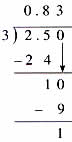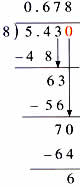Friday , August 19 2022# NCERT 5th Class (CBSE) Mathematics: Decimals

### C. Rounding off Quotients

In this example of division, you can never reach a reminder of zero. In such cases, the quotient is usually rounded.

(a) 2.5÷3 = ?0.83 rounded off to the nearest tenth is 0.8 since the digit in the hundredths place in less than 5.

2.5÷3 = 0.8

(b) 5.43÷8 = ?0.678 rounded to the nearest hundredth is 0.86, since the thousandths place is more than 5.

5.43÷8 = 0.68

(c) 0.75 rounded to the nearest tenth = 0.8

(d) 1.592 rounded to the nearest hundredth = 1.59

### Division by 10, 100, 1000

Look for a pattern. Watch the decimal points.

(a) 821.5÷10 = 82.15

(b) 821.5÷100 = 8.215

(c) 821.5÷1000 = 0.8215

What do you observe?

When dividing by
The decimal point moves to the left by
10
1 decimal place
100
2 decimal place
1000
3 decimal place

Remember this rule by counting the zeros. Move the decimal point to the left as many places as there are zeros.

(d) 4÷10 is the same as 4.0÷10.

So 4÷10 = o.4

(e) 2.35÷100 = 0.0235

## चुनौती हिमालय की 5th NCERT CBSE Hindi Rimjhim Ch 18

चुनौती हिमालय की 5th Class NCERT CBSE Hindi Book Rimjhim Chapter 18 प्रश्न: लद्दाख जम्मू-कश्मीर राज्य में …